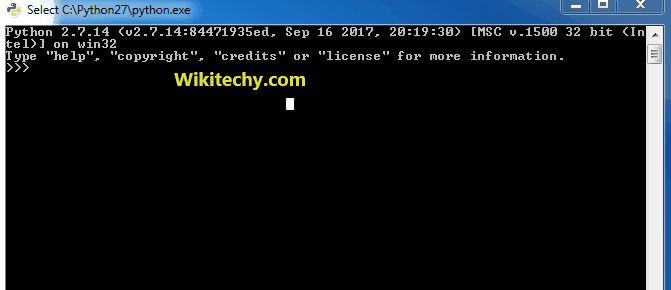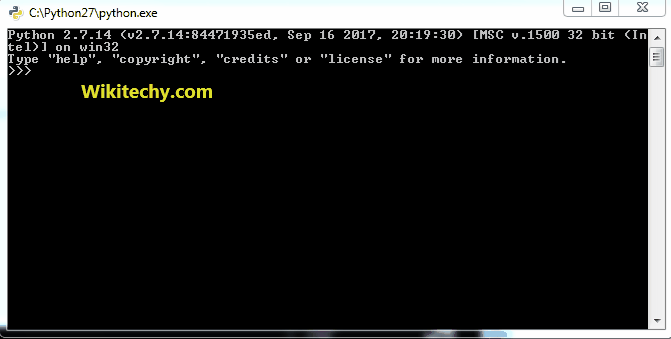# python tutorial - Counters in Python | Accessing Counters - learn python - python programmingLearn Python - Python tutorial - python counters - Python examples - Python programs

Once initialized, counters are accessed just like dictionaries. Also, it does not raise the KeyValue error (if key is not present) instead the value’s count is shown as 0.

Example :

python - Sample - python code :

Output:

```Counter({'blue': 3, 'red': 2, 'yellow': 1})
blue 3
red 2
yellow 1
green 0
```

elements() :
The elements() method returns an iterator that produces all of the items known to the Counter.
Note : Elements with count <= 0 are not included.

Example :

python - Sample - python code :Learn Python - Python tutorial - python accesscounters - Python examples - Python programs

Output :

```Counter({'c': 3, 'b': 2, 'a': 1})
['a', 'b', 'b', 'c', 'c', 'c']
```

most_common() :
most_common() is used to produce a sequence of the n most frequently encountered input values and their respective counts.

python - Sample - python code :

Output :

```f: 219
d: 120
c: 3
```

Wikitechy tutorial site provides you all the learn python , python download , python dictionary , python snake , python list , burmese python , python 3 , python ide , python class , reticulated python , python string , python language , python online , download python , python book , python gui , python for , python threading